#### Trigonometry Homework Help - K-12 Grade Level, College Level Mathematics

Introduction to Trigonometry

The study of triangles and the relationship between their sides and the angles among these sides is known as trigonometry. It is a branch of mathematics that defines the trigonometric functions that defines those relationships and has applicability to the cyclical phenomenon, such as waves. The field evolved during the third century BC as a branch of geometry that is extensively used for astronomical studies.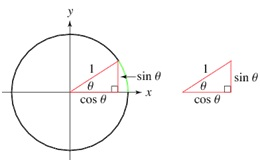Sine

The Sine is a trigonometric function. This is the ratio of the length of the opposite side to the length of the hypotenuse.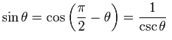Cosine

The Cosine is a trigonometric function. It is the ratio of the length of the adjoining side to the length of the hypotenuse: so called as it is the sine of the complementary or co-angle.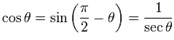Tangent

The Tangent is a trigonometric function. This is the ratio of the length of the opposite side to the length of the adjacent side; so called as it can be stand for by a line segment tangent to the circle which is the line that touches the circle.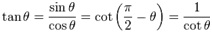Cotangent

Cotangent is the trigonometric function that is for an acute angle and is the ratio between the leg adjacent to the angle whenever it is considered part of a right triangle and the leg opposite.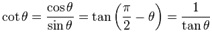Secant:

a trigonometric function sec θ which is reciprocal of the cosine for all real numbers θ for which the cosine is not zero and that is exactly equal to the secant of an angle of measure θ in radians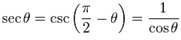Cosecant:

Cosecant is a trigonometric function which is for an acute angle is the ratio between the hypotenuse of a right triangle of which the angle is considered part and the leg opposite the angle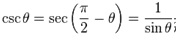Table of Trigonometric functions

 Function Abbreviation Description Identities (using radians) Sine sin opposite / hypotenuseCosine cos adjacent / hypotenuseTangent tan (or tg) opposite / adjacentCotangent cot (or cotan or cotg or ctg or ctn) adjacent / oppositeSecant sec hypotenuse / adjacentCosecant csc (or cosec) hypotenuse / oppositeEmail based Trigonometry Homework Help -Assignment Help

Tutors at the www.tutorsglobe.com are committed to provide the best quality Trigonometry homework help - assignment help. They use their experience, as they have solved thousands of the Trigonometry assignments, which may help you to solve your complex Trigonometry homework. You can find solutions for all the topics come under the trigonometry. The dedicated tutors provide eminence work on your Math homework help and devoted to provide K-12 level math to college level math help before the deadline mentioned by the student. Trigonometry homework help is available here for the students of school, college and university. Tutors Globe assure for the best quality compliance to your homework. Compromise with quality is not in our dictionary. If we feel that we are not able to provide the homework help as per the deadline or given instruction by the student, we refund the money of the student without any delay.

Qualified and Experienced Trigonometry Tutors at www.tutorsglobe.com

Tutors at the www.tutorsglobe.com take pledge to provide full satisfaction and assurance in Trigonometry homework help. Students are getting math homework help services across the globe with 100% satisfaction. We value all our service-users. We provide email based Trigonometry homework help - assignment help. You can join us to ask queries 24x7 with live, experienced and qualified maths tutors specialized in trigonometry.